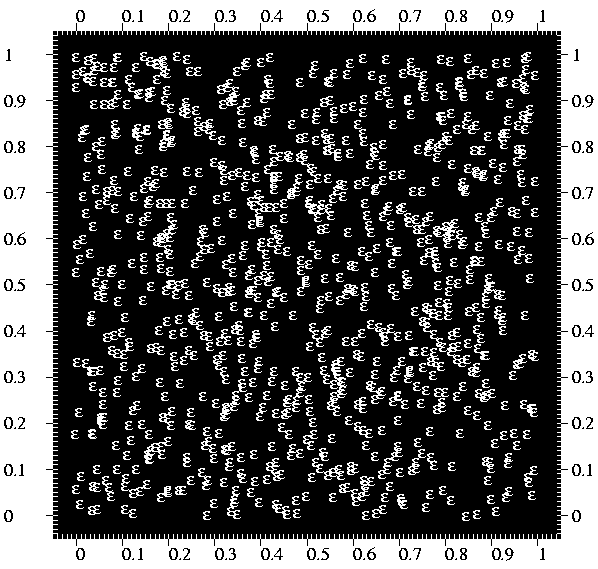# Environment for creative processing of text and numerical data## 999 random points as a scatter plot

K.Vehkalahti (1999)### Edit field:

```   1 *
2 *Assume that SIZE=1800,1800 where plotting area=1400 and edge=200 .
3 *Then set XDIV=edge,area,edge as well as YDIV=edge,area,edge .
4 *Now let m=999 and R=rand(m) . Initialize a (m x 2) null matrix A by
5 *`MAT A=ZER(m,2)` / and generate (pseudo) random observations to it by
6 *`MAT #TRANSFORM A BY R` /
7 *                 Finally plot the observations as a scatter plot by
8 *`PLOT A.MAT 1 2` / using PEN=[Times(14)] and POINT=[WHITE][SYMBOL],e
9 *Other specifications are the following:
10 *HEADER= XLABEL= YLABEL= FRAMES=F F=edge,edge,area,area,-1 FRAME=1
11 *SCALE=-0.05:?_,0(0.1)1,1.05:?_ TICK=[WHITE],0.01,0.01 [FILL-1]=0,0,0,1
12 *DEVICE=PS,EX.PS   XSCALE2=XSCALE YSCALE2=YSCALE TICK2=TICK
13 *

```